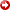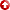[First Hit]# Gassmann With Texture DependentEffectiveGrain Moduli

## Abstract

The use of Gassmann's equation for fluid substitution requires several assumptions, among which is that the rock is composed of a homogeneous matrix. Most reservoir rocks do not conform to this requirement. It is often assumed that the moduli of the matrix components are similar and that a Voigt-Reuss-Hill average can be used to estimate aneffectivegrain modulus. This solution breaks down when the components have very dissimilar moduli. The Brown and Korringa equation is appropriate when the component rock moduli are dissimilar. The problem with this equation is that it replaces Gassmann's grain modulus with solid and porosity related moduli. These parameters are rarely available and are hard to estimate. As a result, it is desirable to use Gassmann's equation whenever possible. The solid bulk modulus in the Brown and Korringa equation tends to be relatively large when the softest component is pore filling and relatively small when it is load bearing. A modification to the Gassmann grain modulus to treat it similar to solid bulk modulus and to calculate theeffectivegrain moduli from weighted average of Hashin-Shtrikman (HS) bounds with the weight dependent on composition and texture. HS bounds can be applied in a two-step workflow: 1.) Calculate bounds oneffectivegrain moduli. 2.) Use fluid moduli and bounds oneffectivegrain moduli to determine bounds for the observed saturated moduli. The workflow's results can be used to quality control the observations and rock petrophysical model. They can also be used determine the weight for theeffectivemoduli in the Gassmann calculation. The range of valid weights for theeffectivegrain moduli can be determined by crossplotting the difference between saturated bounds and observed moduli vs. shale volume. The besteffectivegrain moduli weight for any individual sample is assumed to be the average of the valid bound limits. We assume that the weight is the same for bulk and shear moduli. Final reservoir weights are calculated from best fit to the aggregate of the individual solutions. Shaley sands and sandy shales are likely to have different trends because of the distribution of shale in the rock fabric. In our examples we show that high shale rocks requireeffectivegrain moduli with more weight on the lower bound while low shale rocks require more weight on the upper bound. This is similar to the solid moduli relationship expected from Brown and Korringa equation.